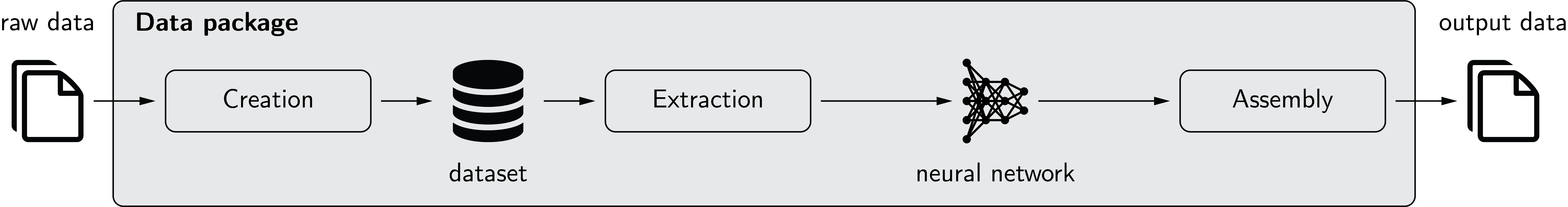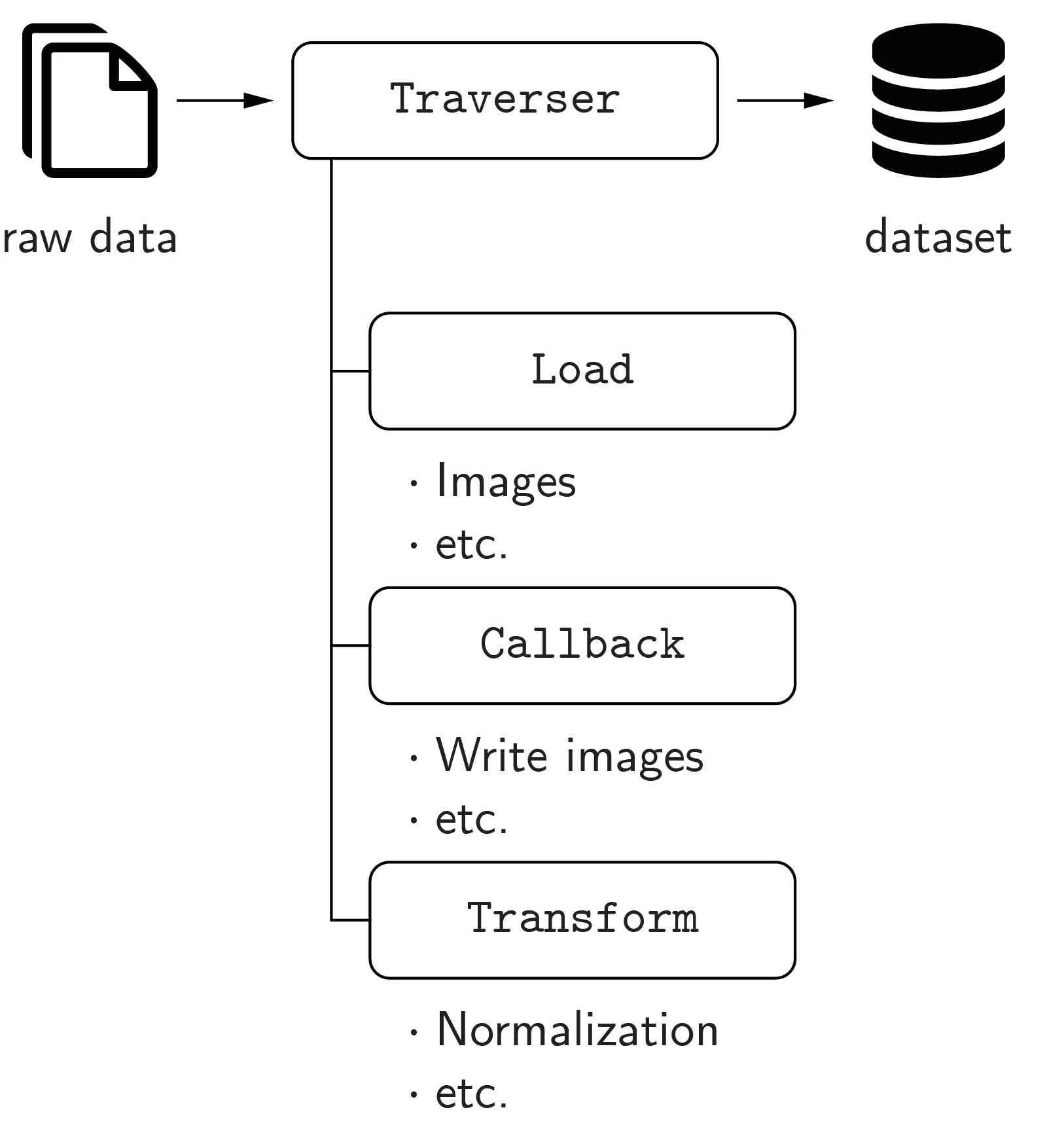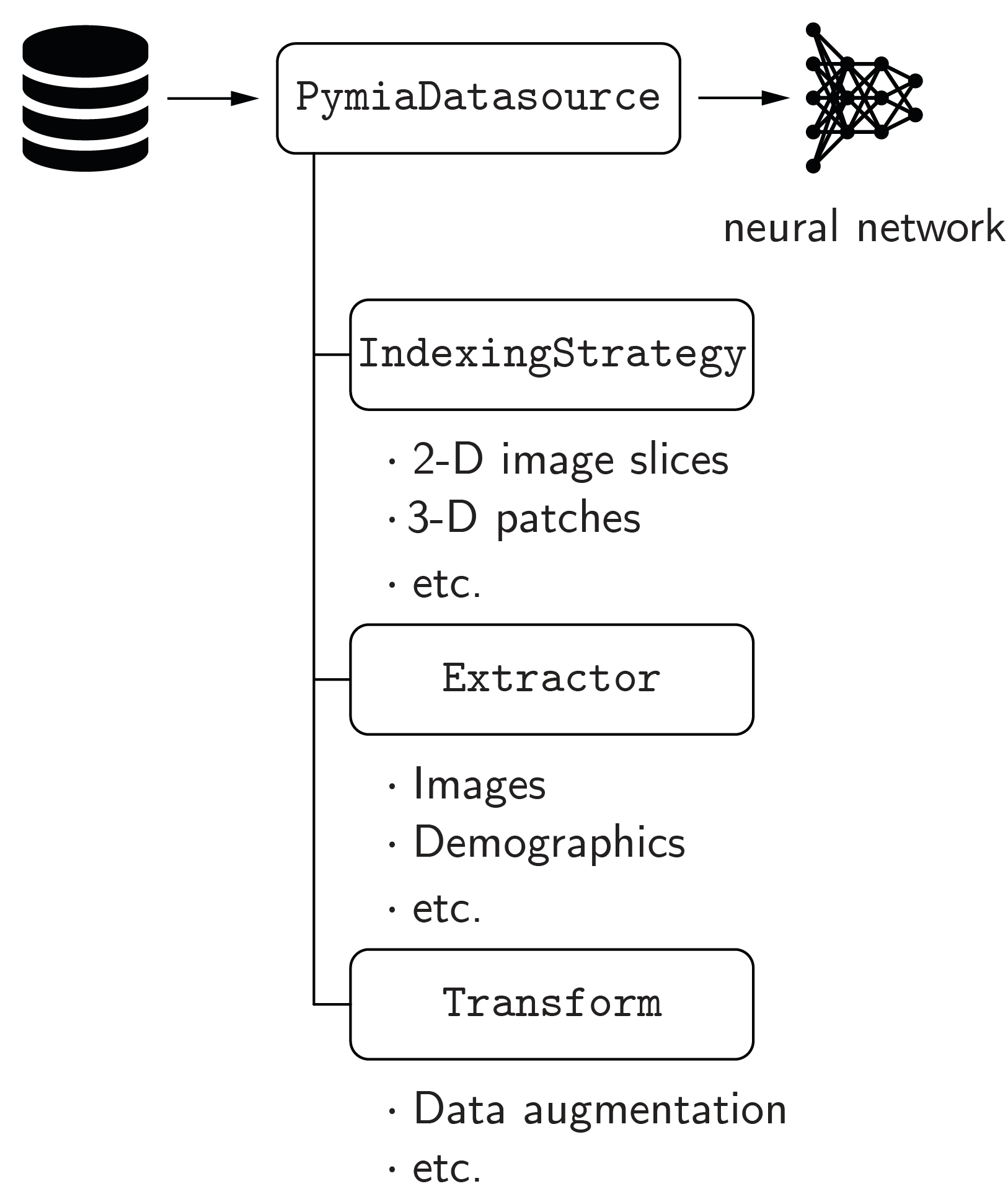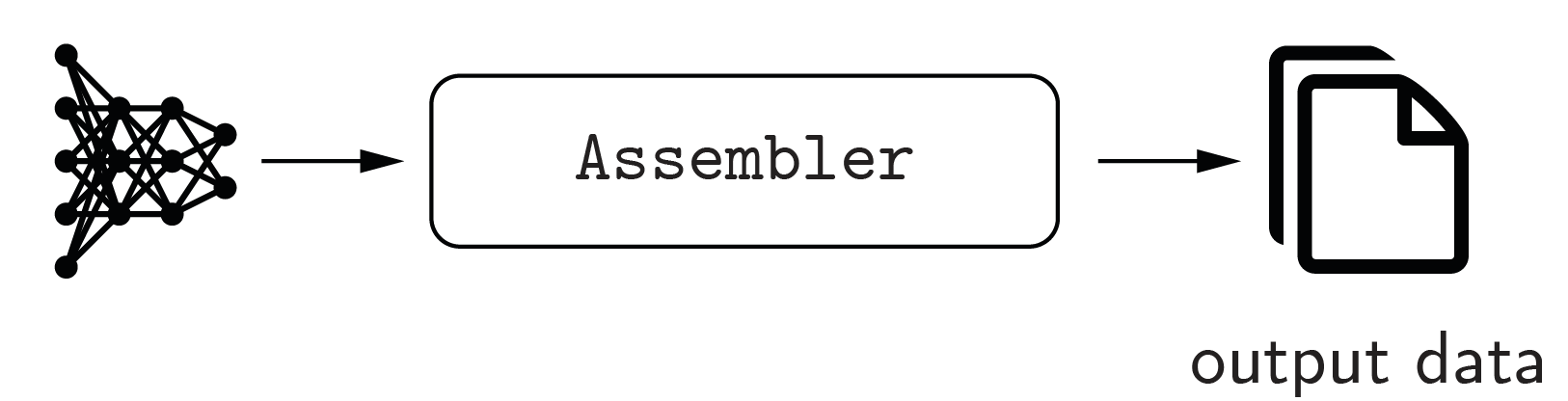# Data (`pymia.data` package)¶

This data package provides data handling functionality for machine learning (especially deep learning) projects. The concept of the data package is illustrated in the figure below.The three main components of the data package are creation, extraction, and assembly.

Creation

The creation of a dataset is managed by the `Traverser` class, which processes the data of every subject (case) iteratively. It employs `Load` and `Callback` classes to load the raw data and write it to the dataset. `Transform` classes can be used to apply modifications to the data, e.g., an intensity normalization. For the ease of usage, the defaults `get_default_callbacks()` and `LoadDefault` are implemented, which cover the most fundamental cases. The code example Creation of a dataset illustrates how to create a dataset.Extraction

Data extraction from the dataset is managed by the `PymiaDatasource` class, which provides a flexible interface for retrieving data, or chunks of data, to form training samples. An `IndexingStrategy` is used to define how the data is indexed, meaning accessing, for instance, an image slice or a 3-D patch of an 3-D image. `Extractor` classes extract the data from the dataset, and `Transform` classes can be used to alter the extracted data. The code example Data extraction and assembly illustrates how to extract data.Assembly

The `Assembler` class manages the assembly of the predicted neural network outputs by using the identical indexing that was employed to extract the data by the `PymiaDatasource` class. The code example Data extraction and assembly illustrates how to assemble data.## Assembler (`pymia.data.assembler` module)¶

class pymia.data.assembler.ApplyTransformInteractionFn(transform: pymia.data.transformation.Transform)[source]
class pymia.data.assembler.AssembleInteractionFn[source]

Bases: `object`

Function interface enabling interaction with the index_expression and the data before it gets added to the assembled prediction in `SubjectAssembler`.

__call__(key, data, index_expr, **kwargs)[source]
Parameters
• key (str) – The identifier or key of the data.

• data (numpy.ndarray) – The data.

• index_expr (.IndexExpression) – The current index_expression that might be modified.

• **kwargs (dict) – Any other arguments

Returns

Modified data and modified index_expression

Return type

tuple

class pymia.data.assembler.Assembler[source]

Bases: `abc.ABC`

Interface for assembling images from batch, which contain parts (chunks) of the images only.

Add the batch results to be assembled.

Parameters
• to_assemble (object, dict) – object or dictionary of objects to be assembled to an image.

• sample_indices (iterable) – iterable of all the sample indices in the processed batch

• last_batch (bool) – Whether the current batch is the last.

abstract get_assembled_subject(subject_index: int)[source]
Parameters

subject_index (int) – Index of the assembled subject to be retrieved.

Returns

The assembled data of the subject (might be multiple arrays).

Return type

object

The indices of the subjects that are finished assembling.

Type

list, set

class pymia.data.assembler.PlaneSubjectAssembler(datasource: pymia.data.extraction.datasource.PymiaDatasource, merge_fn=<function mean_merge_fn>, zero_fn=<function numpy_zeros>)[source]

Assembles predictions of one or multiple subjects where predictions are made in all three planes.

This class assembles the prediction from all planes (axial, coronal, sagittal) and merges the prediction according to `merge_fn`.

Assumes that the network output, i.e. to_assemble, is of shape (B, …, C) where B is the batch size and C is the numbers of channels (must be at least 1) and … refers to an arbitrary image dimension.

Parameters
• datasource (.PymiaDatasource) – The datasource

• merge_fn – A function that processes a sample. Args: planes: list with the assembled prediction for all planes. Returns: Merged numpy.ndarray

• zero_fn – A function that initializes the numpy array to hold the predictions. Args: shape: tuple with the shape of the subject’s labels, id: str identifying the subject. Returns: A np.ndarray

add_batch(to_assemble: Union[numpy.ndarray, Dict[str, numpy.ndarray]], sample_indices: numpy.ndarray, last_batch=False, **kwargs)[source]
get_assembled_subject(subject_index: int)[source]

Assembles predictions of two-dimensional images.

Two-dimensional images do not specifically require assembling. For pipeline compatibility reasons this class provides , nevertheless, a implementation for the two-dimensional case.

Parameters

add_batch(to_assemble: Union[numpy.ndarray, Dict[str, numpy.ndarray]], sample_indices: numpy.ndarray, last_batch=False, **kwargs)[source]
get_assembled_subject(subject_index)[source]
class pymia.data.assembler.SubjectAssembler(datasource: pymia.data.extraction.datasource.PymiaDatasource, zero_fn=<function numpy_zeros>, assemble_interaction_fn=None)[source]

Assembles predictions of one or multiple subjects.

Assumes that the network output, i.e. to_assemble, is of shape (B, …, C) where B is the batch size and C is the numbers of channels (must be at least 1) and … refers to an arbitrary image dimension.

Parameters
• datasource (.PymiaDatasource) – The datasource.

• zero_fn – A function that initializes the numpy array to hold the predictions. Args: shape: tuple with the shape of the subject’s labels. Returns: A np.ndarray

• assemble_interaction_fn (callable, optional) – A callable that may modify the sample and indexing before adding the data to the assembled array. This enables handling special cases. Must follow the `.AssembleInteractionFn.__call__` interface. By default neither data nor indexing is modified.

add_batch(to_assemble: Union[numpy.ndarray, Dict[str, numpy.ndarray]], sample_indices: numpy.ndarray, last_batch=False, **kwargs)[source]

Add the batch results to be assembled.

Parameters
• to_assemble (object, dict) – object or dictionary of objects to be assembled to an image.

• sample_indices (iterable) – iterable of all the sample indices in the processed batch

• last_batch (bool) – Whether the current batch is the last.

get_assembled_subject(subject_index: int)[source]

## Augmentation (`pymia.data.augmentation` module)¶

This module holds classes for data augmentation.

The data augmentation bases on the transformation concept (see `pymia.data.transformation.Transform`) and can easily be incorporated into the data loading process.

The pymia documentation features an code example for Augmentation, which shows how to apply data augmentation in conjunction with the `pymia.data` package. Besides transformations from the `pymia.data.augmentation` module, transformations from the Python packages batchgenerators and TorchIO are integrated.

Warning

The augmentation relies on the random number generator of `numpy`. If you want to obtain reproducible result, set numpy’s seed prior to executing any augmentation:

```>>> import numpy as np
>>> your_seed = 0
>>> np.random.seed(your_seed)
```
class pymia.data.augmentation.RandomCrop(shape: Union[int, tuple], axis: Optional[Union[int, tuple]] = None, p: float = 1.0, entries=('images', 'labels'))[source]

Randomly crops the sample to the specified shape.

The sample shape must be bigger than the crop shape.

Notes

A probability lower than 1.0 might make not much sense because it results in inconsistent output dimensions.

Parameters
• shape (int, tuple) –

The shape of the sample after the cropping. If axis is not defined, the cropping will be applied from the first dimension onwards of the sample. Use None to exclude an axis or define axis to specify the axis/axes to crop. E.g.:

• shape=256 with the default axis parameter results in a shape of 256 x …

• shape=(256, 128) with the default axis parameter results in a shape of 256 x 128 x …

• shape=(None, 256) with the default axis parameter results in a shape of <as before> x 256 x …

• shape=(256, 128) with axis=(1, 0) results in a shape of 128 x 256 x …

• shape=(None, 128, 256) with axis=(1, 2, 0) results in a shape of 256 x <as before> x 256 x …

• axis (int, tuple) – Axis or axes to which the shape int or tuple correspond(s) to. If defined, must have the same length as shape.

• p (float) – The probability of the cropping to be applied.

• entries (tuple) – The sample’s entries to apply the cropping to.

class pymia.data.augmentation.RandomElasticDeformation(num_control_points: int = 4, deformation_sigma: float = 5.0, interpolators: tuple = (3, 1), spatial_rank: int = 2, fill_value: float = 0.0, p: float = 0.5, entries=('images', 'labels'))[source]

Randomly transforms the sample elastically.

Notes

The code bases on NiftyNet’s RandomElasticDeformationLayer class (version 0.3.0).

Warning

Always inspect the results of this transform on some samples (especially for 3-D data).

Parameters
• num_control_points (int) – The number of control points for the b-spline mesh.

• deformation_sigma (float) – The maximum deformation along the deformation mesh.

• interpolators (tuple) – The SimpleITK interpolators to use for each entry in entries.

• spatial_rank (int) – The spatial rank (dimension) of the sample.

• fill_value (float) – The fill value for the resampling.

• p (float) – The probability of the elastic transformation to be applied.

• entries (tuple) – The sample’s entries to apply the elastic transformation to.

class pymia.data.augmentation.RandomMirror(axis: int = - 2, p: float = 1.0, entries=('images', 'labels'))[source]

Randomly mirrors the sample along a given axis.

Parameters
• p (float) – The probability of the mirroring to be applied.

• axis (int) – The axis to apply the mirroring.

• entries (tuple) – The sample’s entries to apply the mirroring to.

class pymia.data.augmentation.RandomRotation90(axes: Tuple[int] = (- 3, - 2), p: float = 1.0, entries=('images', 'labels'))[source]

Randomly rotates the sample 90, 180, or 270 degrees in the plane specified by axes.

Raises

UserWarning – If the plane to rotate is not rectangular.

Parameters
• axes (tuple) – The sample is rotated in the plane defined by the axes. Axes must be of length two and different.

• p (float) – The probability of the rotation to be applied.

• entries (tuple) – The sample’s entries to apply the rotation to.

class pymia.data.augmentation.RandomShift(shift: Union[int, tuple], axis: Optional[Union[int, tuple]] = None, p: float = 1.0, entries=('images', 'labels'))[source]

Randomly shifts the sample along axes by a value from the interval [-p * size(axis), +p * size(axis)], where p is the percentage of shifting and size(axis) is the size along an axis.

Parameters
• shift (int, tuple) –

The percentage of shifting of the axis’ size. If axis is not defined, the shifting will be applied from the first dimension onwards of the sample. Use None to exclude an axis or define axis to specify the axis/axes to crop. E.g.:

• shift=0.2 with the default axis parameter shifts the sample along the 1st axis.

• shift=(0.2, 0.1) with the default axis parameter shifts the sample along the 1st and 2nd axes.

• shift=(None, 0.2) with the default axis parameter shifts the sample along the 2st axis.

• shift=(0.2, 0.1) with axis=(1, 0) shifts the sample along the 1st and 2nd axes.

• shift=(None, 0.1, 0.2) with axis=(1, 2, 0) shifts the sample along the 1st and 3rd axes.

• axis (int, tuple) – Axis or axes to which the shift int or tuple correspond(s) to. If defined, must have the same length as shape.

• p (float) – The probability of the shift to be applied.

• entries (tuple) – The sample’s entries to apply the shifting to.

## Conversion (`pymia.data.conversion` module)¶

This module holds classes related to image conversion.

The main purpose of this module is the conversion between SimpleITK images and numpy arrays.

class pymia.data.conversion.NumpySimpleITKImageBridge[source]

Bases: `object`

A numpy to SimpleITK bridge, which provides static methods to convert between numpy array and SimpleITK image.

static convert(array: numpy.ndarray, properties: pymia.data.conversion.ImageProperties) SimpleITK.SimpleITK.Image[source]

Converts a numpy array to a SimpleITK image.

Parameters
• array (np.ndarray) –

The image as numpy array. The shape can be either:

• shape=(n,), where n = total number of voxels

• shape=(n,v), where n = total number of voxels and v = number of components per pixel (vector image)

• shape=(<reversed image size>), what you get from sitk.GetArrayFromImage()

• shape=(<reversed image size>,v), what you get from sitk.GetArrayFromImage()

and v = number of components per pixel (vector image)

• properties (ImageProperties) – The image properties.

Returns

The SimpleITK image.

Return type

sitk.Image

class pymia.data.conversion.SimpleITKNumpyImageBridge[source]

Bases: `object`

A SimpleITK to numpy bridge.

Converts SimpleITK images to numpy arrays. Use the `NumpySimpleITKImageBridge` to convert back.

static convert(image: SimpleITK.SimpleITK.Image) Tuple[numpy.ndarray, pymia.data.conversion.ImageProperties][source]

Converts an image to a numpy array and an ImageProperties class.

Parameters

image (SimpleITK.Image) – The image.

Returns

The image as numpy array and the image properties.

Return type

A Tuple[np.ndarray, ImageProperties]

Raises

ValueError – If image is None.

## Definition (`pymia.data.definition` module)¶

This module contains global definitions for the `pymia.data` package.

pymia.data.definition.KEY_CATEGORIES = 'categories'
pymia.data.definition.KEY_FILE_ROOT = 'file_root'
pymia.data.definition.KEY_IMAGES = 'images'
pymia.data.definition.KEY_INDEX_EXPR = 'index_expr'
pymia.data.definition.KEY_LABELS = 'labels'
pymia.data.definition.KEY_PLACEHOLDER_FILES = '{}_files'
pymia.data.definition.KEY_PLACEHOLDER_NAMES = '{}_names'
pymia.data.definition.KEY_PLACEHOLDER_NAMES_SELECTED = '{}_names_selected'
pymia.data.definition.KEY_PLACEHOLDER_PROPERTIES = '{}_properties'
pymia.data.definition.KEY_PROPERTIES = 'properties'
pymia.data.definition.KEY_SAMPLE_INDEX = 'sample_index'
pymia.data.definition.KEY_SHAPE = 'shape'
pymia.data.definition.KEY_SUBJECT = 'subject'
pymia.data.definition.KEY_SUBJECT_FILES = 'subject_files'
pymia.data.definition.KEY_SUBJECT_INDEX = 'subject_index'

## Index expression (`pymia.data.indexexpression` module)¶

class pymia.data.indexexpression.IndexExpression(indexing: Optional[Union[int, tuple, List[int], List[tuple], List[list]]] = None, axis: Optional[Union[int, tuple]] = None)[source]

Bases: `object`

Defines the indexing of a chunk of raw data in the dataset.

Parameters
• indexing (int, tuple, list) – The indexing. If `int` or list of `int`, individual entries of and axis are indexed. If `tuple` or list of `tuple`, the axis should be sliced.

• axis (int, tuple) – The axis/axes to the corresponding indexing. If `tuple`, the length has to be equal to the list length of `indexing`

expression

list of `slice` objects defining the slicing each axis

get_indexing()[source]
Returns

a list tuples defining the indexing (i.e., None, index, (start, stop)) at each axis. Can be used to generate a new index expression.

Return type

list

set_indexing(indexing: Union[int, tuple, slice, List[int], List[tuple], List[list]], axis: Optional[Union[int, tuple]] = None)[source]

## Subject file (`pymia.data.subjectfile` module)¶

class pymia.data.subjectfile.FileCategory(entries=None)[source]

Bases: `object`

class pymia.data.subjectfile.SubjectFile(subject: str, **file_groups)[source]

Bases: `object`

Holds the file information of a subject.

Parameters
• subject (str) – The subject identifier.

• **file_groups (dict) – The groups of file types containing the file path entries.

get_all_files()[source]
Returns

All file path entries of a subject flattened (without groups/category).

Return type

dict

## Transformation (`pymia.data.transformation` module)¶

class pymia.data.transformation.ClipPercentile(upper_percentile: float, lower_percentile: Optional[float] = None, loop_axis=None, entries=('images',))[source]
transform_entry(np_entry, entry, loop_i=None) numpy.ndarray[source]
class pymia.data.transformation.ComposeTransform(transforms: Iterable[pymia.data.transformation.Transform])[source]
class pymia.data.transformation.IntensityNormalization(loop_axis=None, entries=('images',))[source]
transform_entry(np_entry, entry, loop_i=None) numpy.ndarray[source]
class pymia.data.transformation.IntensityRescale(lower, upper, loop_axis=None, entries=('images',))[source]
transform_entry(np_entry, entry, loop_i=None) numpy.ndarray[source]
class pymia.data.transformation.LambdaTransform(lambda_fn, loop_axis=None, entries=('images',))[source]
transform_entry(np_entry, entry, loop_i=None) numpy.ndarray[source]
class pymia.data.transformation.LoopEntryTransform(loop_axis=None, entries=())[source]

Bases: `pymia.data.transformation.Transform`, `abc.ABC`

static loop_entries(sample: dict, fn, entries, loop_axis=None)[source]
abstract transform_entry(np_entry, entry, loop_i=None) numpy.ndarray[source]
class pymia.data.transformation.Permute(permutation: tuple, entries=('images', 'labels'))[source]
transform_entry(np_entry, entry, loop_i=None) numpy.ndarray[source]
class pymia.data.transformation.RandomCrop(size: tuple, loop_axis=None, entries=('images', 'labels'))[source]
transform_entry(np_entry, entry, loop_i=None) numpy.ndarray[source]
class pymia.data.transformation.Relabel(label_changes: Dict[int, int], entries=('labels',))[source]
transform_entry(np_entry, entry, loop_i=None) numpy.ndarray[source]
class pymia.data.transformation.Reshape(shapes: dict)[source]

Initializes a new instance of the Reshape class.

Parameters

shapes (dict) – A dict with keys being the entries and the values the new shapes of the entries. E.g. shapes = {defs.KEY_IMAGES: (-1, 4), defs.KEY_LABELS : (-1, 1)}

transform_entry(np_entry, entry, loop_i=None) numpy.ndarray[source]
class pymia.data.transformation.SizeCorrection(shape: Tuple[Union[None, int], ...], pad_value: int = 0, entries=('images', 'labels'))[source]

Size correction transformation.

Corrects the size, i.e. shape, of an array to a given reference shape.

Initializes a new instance of the SizeCorrection class.

Parameters
• shape (tuple of ints) – The reference shape in NumPy format, i.e. z-, y-, x-order. To not correct an axis dimension, set the axis value to None.

• pad_value (int) – The value to set the padded values of the array.

• () (entries) –

class pymia.data.transformation.Squeeze(entries=('images', 'labels'), squeeze_axis=None)[source]
transform_entry(np_entry, entry, loop_i=None) numpy.ndarray[source]
class pymia.data.transformation.Transform[source]

Bases: `abc.ABC`

class pymia.data.transformation.UnSqueeze(axis=- 1, entries=('images', 'labels'))[source]
transform_entry(np_entry, entry, loop_i=None) numpy.ndarray[source]
pymia.data.transformation.check_and_return(obj, type_)[source]